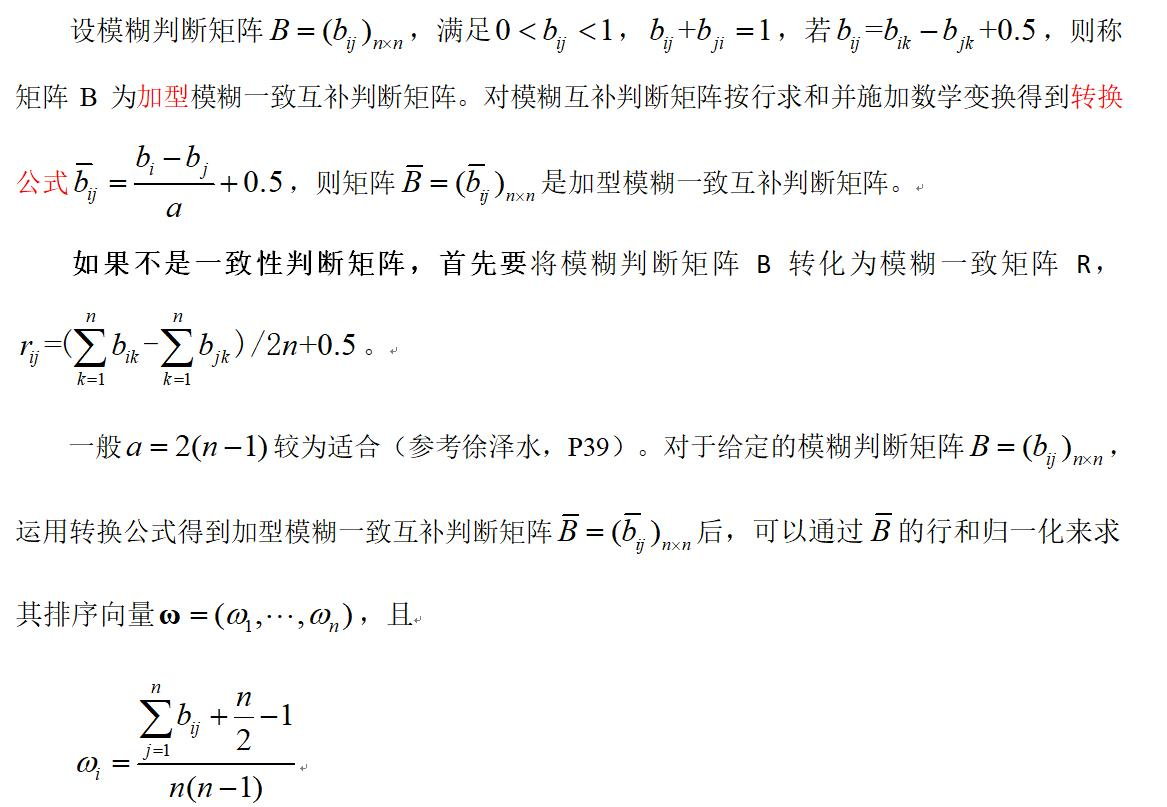• 层次分析法中判断矩阵的填写方法、一致性检验的步骤、以及根据判断矩阵计算权重的方法

目录：
准则层判断矩阵怎么填写方案层判断矩阵怎么填写关于判断矩阵和一致矩阵的知识点补充一致性检验的步骤怎样通过判断矩阵去计算权重（三种方法），及相应的代码示例

准则层判断矩阵的填写：
填写准则层判断矩阵的目的是确定各准则（指标）所占的比重，填写好层次分析表的指标权重列，例如在选择最佳旅游地问题的指标景色、花费、居住、饮食、交通各自占比是多少，后续可以通过这些指标占比计算出每一个可选方案的总分。填表的方法是依据标度表，两两比较指标的重要程度，只需要比较10次就可以完成准则层判断矩阵的填写

方案层判断矩阵的填写
填写方案层判断矩阵的目的是给出，对于某一特定指标，它在各个可选方案的具体得分是多少，也就是给出层次分析表的每一横行的数据。方法是依据标度表，填写好判断矩阵。有几个评价指标，就需要填多少此方案层判断矩阵。知识点补充：
判断矩阵（正互反矩阵）
首先判断矩阵一定是一个方阵判断矩阵每一个数据 Aij表示与指标 j相比 i的重要程度当 i=j 时，两个指标相同，因此同等重要，记为1，因此判断矩阵的对角线元素为1每一个元素均大于零，且 Aij * Aji=1
在层次分析法中，我们构造的矩阵的均为判断矩阵
一致矩阵
矩阵首先满足判断矩阵的所有特点若判断矩阵满足 Aij * Ajk = Aik,直观的看就是矩阵的各行（各列）成倍数关系
注意点：在使用判断矩阵求权重之前，必须对其进行一致性检验

一致性检验的步骤：
第一步：计算一致性指标CI

C

I

=

λ

max

⁡

−

n

n

−

1

CI\,\,=\,\,\frac{\lambda _{\max}-n}{n-1}

第二步：查找对应的平均随机一致性指标RI第三步：计算一致性比例CR

C

R

=

C

I

R

I

CR\,\,=\,\,\frac{CI}{RI}

判断：如果CR<0.1,则可认为判断举证的一致性可以接受；否则需要对判断矩阵进行修改
一致性检验的MATLAB代码如下：
disp('请输入判断矩阵A')
A=input('A=');
[n,n] = size(A);
[V,D] = eig(A);%求出矩阵A的特征值和特征向量
Max_eig = max(max(D));%找到矩阵A的最大特征值
% 下面是计算一致性比例CR的环节 %
CI = (Max_eig - n) / (n-1);
RI=[0 0.0001 0.52 0.89 1.12 1.26 1.36 1.41 1.46 1.49 1.52 1.54 1.56 1.58 1.59];
%注意哦，这里的RI最多支持 n = 15
% 这里n=2时，一定是一致矩阵，所以CI = 0，我们为了避免分母为0，将这里的第二个元素改为了很接近0的正数
CR=CI/RI(n);
disp('一致性指标CI=');disp(CI);
disp('一致性比例CR=');disp(CR);
if CR<0.10
disp('因为CR<0.10，所以该判断矩阵A的一致性可以接受!');
else
disp('注意：CR >= 0.10，因此该判断矩阵A需要进行修改!');
end



通过判断矩阵求权重
方法一、算数平均法求权重
第一步：将判断矩阵按照列归一化（每一个元素除以器所在列的和）
第二步：将归一化的各列相加（按行求和）
第三步：将相加后得到的向量中的每个元素除以n即可得到权重向量
具体数学表达：
假设判断矩阵为下面这个矩阵A：

A

=

[

a

11

a

12

⋯

a

1

n

a

21

a

22

⋯

a

2

n

⋮

⋮

⋱

⋮

a

n

1

a

n

2

⋯

a

n

n

]

A=\left[ \begin{matrix} a_{11}& a_{12}& \cdots& a_{1n}\\ a_{21}& a_{22}& \cdots& a_{2n}\\ \vdots& \vdots& \ddots& \vdots\\ a_{n1}& a_{n2}& \cdots& a_{nn}\\ \end{matrix} \right]

那么算数平均法求得的权重向量为：

w

i

=

1

n

∑

j

=

1

n

a

i

j

∑

k

=

1

n

a

k

j

w_i=\frac{1}{n}\sum_{j=1}^n{\frac{a_{ij}}{\sum_{k=1}^n{a_{kj}}}}\,\,

MATLAB代码如下：
disp('请输入判断矩阵A')
A=input('A=');
[n,n] = size(A);

Sum_A = sum(A);   %sum函数默认是对矩阵的每一列进行累加，即按行求和
SUM_A = repmat(Sum_A,n,1);    %将Sum_A这个行向量，重复n行，重复一列
Stand_A = A ./ SUM_A;         %将矩阵A归一化，即每一个元素除以其所在列的和

disp('算术平均法求权重的结果为：');
disp(sum(Stand_A,2)./n)      %把归一化的矩阵的每一行累加，然后除以n,得到权重

方法二、几何平均法求权重
第一步：将A元素按照行相乘得到一个新的列向量
第二步：将新的列向量的每个分量开n次方
第三步：对该列向量进行归一化即可得到权重向量
假设判断矩阵为下面这个矩阵A：

A

=

[

a

11

a

12

⋯

a

1

n

a

21

a

22

⋯

a

2

n

⋮

⋮

⋱

⋮

a

n

1

a

n

2

⋯

a

n

n

]

A=\left[ \begin{matrix} a_{11}& a_{12}& \cdots& a_{1n}\\ a_{21}& a_{22}& \cdots& a_{2n}\\ \vdots& \vdots& \ddots& \vdots\\ a_{n1}& a_{n2}& \cdots& a_{nn}\\ \end{matrix} \right]

那么几何平均法求得的权重向量为：

w

i

=

(

∏

j

=

1

n

a

i

j

)

1

n

∑

k

=

1

n

(

∏

j

=

1

n

a

k

j

)

1

n

,

(

i

=

1

,

2

,

…

，

n

)

w_i=\frac{\left( \prod_{j=1}^n{a_{ij}} \right) ^{\frac{1}{n}}}{\sum_{k=1}^n{\left( \prod_{j=1}^n{a_{kj}} \right) ^{\frac{1}{n}}}},\left( i=1,2,…\text{，}n \right)

注意：每一种方法求得的权重和应该为1，由于四舍五入导致的误差可以忽略，一般结果保留四位小数
MATLAB代码如下：
disp('请输入判断矩阵A')
A=input('A=');
[n,n] = size(A);  %获得矩阵A的行和列的大小

Prduct_A = prod(A,2);       %把矩阵A的每一行累乘，即按照列累乘
Prduct_n_A = Prduct_A .^ (1/n);   %将新的列向量的每个分量开n次方
disp('几何平均法求权重的结果为：');
disp(Prduct_n_A ./ sum(Prduct_n_A))  %对该列向量进行归一化即可得到权重向量

方法三、特征值法求权重（常用）
知识点提醒：一致矩阵有一个特征值为n,其余特征值均为0，并且当矩阵的特征值为n时，其对应的特征向量为

k

[

1

a

11

,

1

a

12

,

…

,

1

a

1

n

]

T

,

(

k

≠

0

)

k\left[ \frac{1}{a_{11}},\frac{1}{a_{12}},…,\frac{1}{a_{1n}} \right] ^T,\left( k\ne 0 \right)

第一步：求出矩阵A的最大特征值和以及其对应的特征向量
第二步：对求出的特征向量进行归一化即可得到所求的权重
MATLAB代码如下：
disp('请输入判断矩阵A')
A=input('A=');

%求矩阵A的全部特征值，构成对角阵D，求A的特征向量构成V的列向量（V的每一列都是D中与之相同列的特征值的特征向量）
[V,D] = eig(A);
Max_eig = max(max(D));   %求出矩阵A的最大的特征值
[r,c]=find(D == Max_eig , 1);  %返回最大特征值所在的行和列，其中C记录所在列
disp('特征值法求权重的结果为：');
disp( V(:,c) ./ sum(V(:,c)) )  %对最大特征值对应的特征向量进行归一化处理

友情提示：在比赛当中，建议三种方法全部列出来，但仅适用特征值法求得的权重结果进行计算

更多有关与层次分析法的经典获奖论文，以及建模论文模板等资料关注公众号，回复，“层次分析法”，即可免费领取！！！展开全文• 引进了互反判断矩阵与互补判断矩阵之间的转换公式，介绍了完全一致性互反判断矩阵和完全一致性互补判断矩阵之间的关系。提出了3种基于互反判断矩阵的互补判断矩阵排序方法，详细地研究了它们的一些优良性质，如：强...
• 利用粒子群算法计算AHP判断矩阵排序权重.pdf
• 对区间数服从不同概率分布时所产生的不确定度进行了评定，结合误差传递理论，提出了计算区间数判断矩阵权重的一种新方法。
• 对于matlab新手而言，主要在判断矩阵未通过一致性检验的时候，需要重新输入，重新计算。 clc; clear;% 清除所有命令窗口，清除所有变量 while true %无条件进入循环 A=input('请输入判断矩阵A='); [m,n]=size(A); %...
层次分析法原理简单，matlab实现起来也较容易。 对于matlab新手而言，主要在判断矩阵未通过一致性检验的时候，需要重新输入，重新计算。
clc;
clear;% 清除所有命令窗口，清除所有变量
while true %无条件进入循环
A=input('请输入判断矩阵A=');
[m,n]=size(A);                     %获取指标个数
RI=[  0	 0	 0.58 	0.90	1.12	1.26	1.36	1.41	1.46];
[V,D]=eig(A);                      %求判断矩阵的特征值和特征向量，V特征值，D特征向量；
tz=max(D);
B=max(tz);                         %最大特征值
[row, col]=find(D==B);             %最大特征值所在位置
C=V(:,col);                        %对应特征向量
CI=(B-n)/(n-1);                    %计算一致性检验指标CI
CR=CI/RI(1,n);
if CR<0.10
disp('CI=');disp(CI);
disp('CR=');disp(CR);
disp('对比矩阵A通过一致性检验，各向量权重向量Q为：');
break;

else
disp('对比矩阵A未通过一致性检验，需对对比矩阵A重新构造');
continue;
end

end

Q=zeros(n,1);
for i=1:n
Q(i,1)=C(i,1)/sum(C(:,1)); %特征向量标准化
end
Q  %最后输出权重值

展开全文• 计算与群组判断矩阵差异最小的最优可能度矩阵和无约束的最优满意度矩阵; 采用两个最优矩阵上三角元素的几何 平均, 获取满足最优可能满意度的群决策合成矩阵. 最后通过算例表明了所提出方法的有效性和实用性.</p>
• 讲解了如何利用Matlab求解层次分析法中判断矩阵的权向量，并进行一致性判断。
• 决策者利用一定的标度对属性进行两两比较，并构造判断矩阵，然后按一定的排序方法计算判断矩阵的排序向量，从而获得属性权重，最后在根据各种算子进行多属性群决策。 （一）模糊互补判断矩阵排序法 1.加型模糊互补...
决策者利用一定的标度对属性进行两两比较，并构造判断矩阵，然后按一定的排序方法计算判断矩阵的排序向量，从而获得属性权重，最后在根据各种算子进行多属性群决策。
（一）模糊互补判断矩阵排序法
1.加型模糊互补判断矩阵排序的中转法 2.乘型模糊互补判断矩阵排序的和积法
（二）模糊互补判断矩阵的最优化排序方法

加型模糊互补判断矩阵排序的最小方差法
乘型模糊互补判断矩阵排序的最小平方法
模糊互补判断矩阵排序的幂法

（一）模糊互补判断矩阵排序法 4. 加型模糊互补判断矩阵排序的中转法 判断矩阵的标度和含义如下表所示： 标度 含义 0.1 极端不重要（前比后） 0.3 明显不重要 0.5 同等重要 0.7 明显重要 0.9 极端重要 按上述标度构成判断矩阵，bii=0.5，也满足其他条件。此方法称为模糊互补判断矩阵排序的中转法（MTM）。 特点：该方法得到的排序向量的分量之间的差异较小，有时不易区分。 2.乘型模糊互补判断矩阵排序的和积法 
展开全文matlab 算法 线性代数 动态规划
• 论文研究- 群体判断矩阵及权向量的最优传递矩阵求法.pdf, 层次分析法的关键步骤是构造判断矩阵,判断矩阵一经确定,即可选用各种各样的计算方法得到判断矩阵的排序权值,如可选用行和正规化方法、列和求逆法、和积法、...
• 可在MATLAB中进行判断矩阵的权重计算，包括算术平均值法，特征值法，并进行一致性检验，亲测可用
• 论文研究-判断矩阵一致性修正的新方法.pdf, 提出了判断矩阵一致性修正的一种新方法 ,并给出了其简洁、实用的迭代算法 .该法利用判断矩阵的特征向量对矩阵中偏差最大的列...
• ## MATLAB判断矩阵相等

千次阅读 2014-11-26 01:27:44
1. A==B;%得到的是一个矩阵，对应值相等则返回1，否则返回0 ...%原理和2相同，但是计算速度要快于2数倍 4. isequal(A,B);%相等返回1，否则返回0;支持元胞矩阵，其他的函数基本不支持 4. 若不要求包含元素顺序必
1. A==B;%得到的是一个矩阵，对应值相等则返回1，否则返回0
2. ~norm(A-B);%若A==B则A-B全零，norm(A-B)的结果为0，否则为1；其中norm为范数
3. ~sum(sum(abs(A-B)));%原理和2相同，但是计算速度要快于2数倍
4. isequal(A,B);%相等返回1，否则返回0;支持元胞矩阵，其他的函数基本不支持 4. 若不要求包含元素顺序必须相同，还可以使用ismember，setdiff，setxor等
展开全文matlab
• 给出了满意一致性指标的计算方法, 通过该方法可以找出语言判断矩阵中所有不合逻辑的判断元素组,判定语言判断矩阵的满意一致性程度, 有效地解决存在两个方案无差异的语言判断矩阵的满意一致性的判定问题; 最后通过两...
• 针对基于不同粒度语言判断...采用OWA算子将每个决策者给出语言判断矩阵所对应的互补判断矩阵集结为群的偏好矩阵，并通过计算每个方案的优势度来确定最优方案；最后给出一个算例说明了所提出的方法的有效性与实用性。
• 针对基于语言判断矩阵的群决策问题，提出了一种基于语言信息处理的群决策方法。在描述了基于语言判断矩阵的群决策问题后，通过变量转换关系对语言短语集中的有序语言短语进行"量化"，构造了与决策者给出语言判断矩阵...
• 层次分析法matlab源程序，用于一致性检验，特征值、特征向量计算
• 今天小编就为大家分享一篇python 判断矩阵中每行非零个数的方法，具有很好的参考价值，希望对大家有所帮助。一起跟随小编过来看看吧
• 提出“差标度法”合理确定标度，用这种方法构造的判断矩阵在不违背人的主观意愿的前提下一次满足一致性，极大地简化了计算过程，打破了以往纯粹从数学的角度来追求一致性的标度研究模式，扩展且灵活运用了萨蒂的9...
• 针对应用层次分析法时，对判断矩阵现有调整方法存的不足，提出一种新的优化调整算法，计算 判断矩阵元素各个位置 上能达到最佳一致性时的元素值，通过原判断矩阵元素和...
• disp('请输入判断矩阵A') A=input('A='); [n,n] = size(A) %方法1： 算术平均法 Sum_A = sum(A); SUM_A = repmat(Sum_A,n,1); Stand_A = A ./ SUM_A; Stand_A = A ./ Sum_A; % 这样也可以的 disp('算术平均法求权重...源程序代码 一致性检验
• 该方法利用判断矩阵的特征值及其Frobenius范数,给出判断矩阵的可能度和满意度的定义与计算公式,分别考察一致性改进程度和相对原始判断矩阵的偏离程度,并将二者合并为一个衡量一致性改善效果的综合指标:判断矩阵的...
• 文章提出了区间数判断矩阵的准一致性概念作为一个新的评判标准，并在此基础上对已较为广泛应用的计算区间数判断矩阵权重向量的IEM算法进行了修正，给出了一个可通用的计算权重向量的公式，并举例证明了该方法的合理...
• 为修正AHP中判断矩阵的一致性,提出了用加速遗传算法同时修正判断矩阵一致性和计算AHP中各要素的排序权值的新方法(AGA-CAHP).理论分析和实例分析的初步结果说明:AGA-CAHP法直观、实用,计算结果稳定、精度高,在系统...
• 不过，对于小矩阵来说，检查矩阵是否为对称正定矩阵的这两种方法的计算时间之间的差异可以忽略。 此方法要求您在执行测试之前，先使用 issymmetric 来检查矩阵是否对称（如果矩阵不对称，则不需要计算特征值）。 tf ...
• 奇异矩阵判断方法 首先，看这个矩阵是不是方阵（即行数和列数相等的矩阵。 … 然后，再看此矩阵的行列式|A|是否等于0，若等于0，称矩阵A为奇异矩阵；若不等于0，称矩阵A为非奇异矩阵。 求矩阵行列式 d = det(A) det......